# Viterbi Decoder

Decode convolutionally encoded data using Viterbi algorithm

•Libraries:
Communications Toolbox / Error Detection and Correction / Convolutional
Communications Toolbox HDL Support / Error Detection and Correction / Convolutional

## Description

The Viterbi Decoder block decodes convolutionally encoded input symbols to produce binary output symbols by using the Viterbi algorithm. A trellis structure specifies the convolutional encoding scheme. For more information, see Trellis Description of a Convolutional Code.

This block can process several symbols at a time for faster performance and can accept inputs that vary in length during simulation. For more information about variable-size signals, see Variable-Size Signal Basics (Simulink).

This icon shows all the optional block ports enabled.## Examples

expand all

Apply convolutional encoding and BPSK modulation to a binary signal, pass the modulated signal through an AWGN channel. Compute the symbol error rate (SER) of the signal after applying BPSK demodulation and Viterbi decoding.

Explore model

The doc_conv model generates a binary signal by using a Bernoulli Binary Generator block. The Convolutional Encoder block encodes the signal. The BPSK Modulator Baseband block modulates the signal. The AWGN Channel block adds noise to the signal. To demodulate the BPSK modulated signal with zero phase shift, simply extract the value of the real component of the complex symbol demodulation by using the Complex to Real-Imag (Simulink) block. The Viterbi Decoder block decodes the signal. The Error Rate Calculation block computes the SER.Run simulation

ans = 'Filtering the signal through an AWGN channel with the EsN0 set to -1 dB, the computed SER is 0.005608.' ans = 'For 53499 transmitted symbols, there were 300 symbols errors.' 

This example simulates a punctured coding system that uses rate 1/2 convolutional encoding and Viterbi decoding. The complexity of a Viterbi decoder increases rapidly with the code rate. The puncturing technique enables encoding and decoding of higher rate codes by using standard lower rate coders.

The cm_punct_conv_code model transmits a convolutionally encoded BPSK signal through an AWGN channel, demodulates the received signal, and then performs Viterbi decoding to recover the uncoded signal. To compute the error rate, the model compares the original % signal and the decoded signal.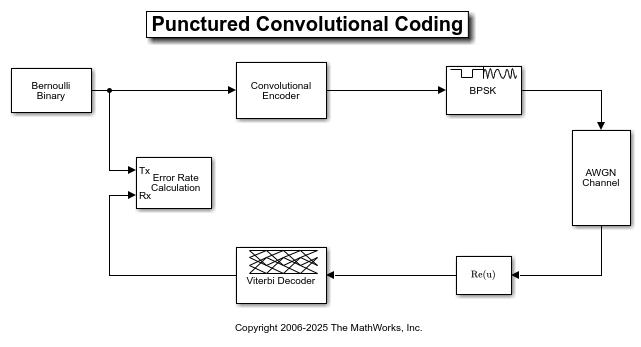The model uses the PreloadFcn callback function to set these workspace variables for initialization of block parameters:

puncvec = [1;1;0;1;1;0]; EsN0dB = 2; traceback = 96; % Viterbi traceback depth

The blocks in this model perform these operations:

• Bernoulli Binary Generator — Sets Sample per frames to 3. The block creates a sequence of random bits outputting three samples per frame at each sample time.

• Convolutional Encoder — Uses the default setting for Trellis structure, selects Puncture code, and sets Puncture vector to the workspace variable puncvec. The block encodes frames of data by puncturing a rate 1/2, constraint length 7 convolutional code to a rate 3/4 code. The puncture vector specified by puncvec is the optimal puncture vector for the rate 1/2, constraint length 7 convolutional code. A 1 in the puncture vector indicates that the bit in the corresponding position of the coded vector is sent to the output vector, while a 0 indicates that the bit is removed. For the configured encoder, coded bits in positions 1, 2, 4, and 5 are transmitted, while bits in positions 3 and 6 are removed. The rate 3/4 code means that for every 3 bits of input, the punctured code generates 4 bits of output.

• BPSK Modulator Baseband — Modulates the encoded message using default parameter values.

• AWGN Channel — Sets Mode to Signal to noise ratio (Es/No) and sets Es/No (dB) to the workspace variable EsN0dB. Since the modulator block generates unit power signals, Input signal power, referenced to 1 ohm (watts) keeps its default value of 1.

• Viterbi Decoder — Uses settings for Trellis structure, Punctured code, and Puncture vector that align with the Convolutional Encoder block. The block sets Decision type to Unquantized and Traceback depth to the traceback workspace variable. To decode the specified convolutional code without puncturing the code, a traceback depth of 40 is sufficient. However, to give the decoder enough data to resolve the ambiguities introduced by the punctures, the block uses a traceback depth of 96 to decode the punctured code. Similar to the convolutional encoder, the puncture vector for the decoder indicates the locations of the punctures. For the decoder, the locations are bits to ignore in the decoding process because the punctured bits are not transmitted and there is no information to indicate their values. Each 1 in the puncture vector indicates a transmitted bit, and each 0 indicates a punctured bit to ignore in the input to the decoder.

• Complex to Real-Imag (Simulink) — Demodulates the BPSK signal by extracting the real part of the complex samples.

• Error Rate Calculation — Uses the Receive delay value to account for total number of samples of system delay and compares the decoded bits to the original source bits. The block outputs a three-element vector containing the calculated BER, the number of errors observed, and the number of bits processed. The Receive delay is set to the traceback workspace variable because the Viterbi traceback depth causes the only delay in the system. Typically, BER simulations run until a minimum number of errors have occurred, or until the simulation processes a maximum number of bits. The Error Rate Calculation block selects the Stop simulation parameter and sets the target number of errors to 100 and the maximum number of symbols to 1e6 to control the duration of the simulation.

Evaluate Bit Error Rate

Generate a bit error rate curve by running this code to simulate the model over a range of EbN0 settings.

Compare the simulation results with an approximation of the bit error probability bound for a punctured code as per [ 1 ]. The bit error rate performance of a rate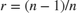punctured code is bounded above by the expression:In this expression, erfc denotes the complementary error function,is the code rate, and bothandare dependent on the particular code. For the rate 3/4 code of this example,= 5,= 42,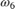= 201,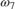= 1492, and so on. For more information, see reference [ 1 ].

Compute an approximation of the theoretical bound using the first seven terms of the summation for Eb/N0 values in 2:0.02:5. The values used for nerr come from reference [ 2 ], Table II.

Plot the simulation results, a fitted curve, and theoretical bounds.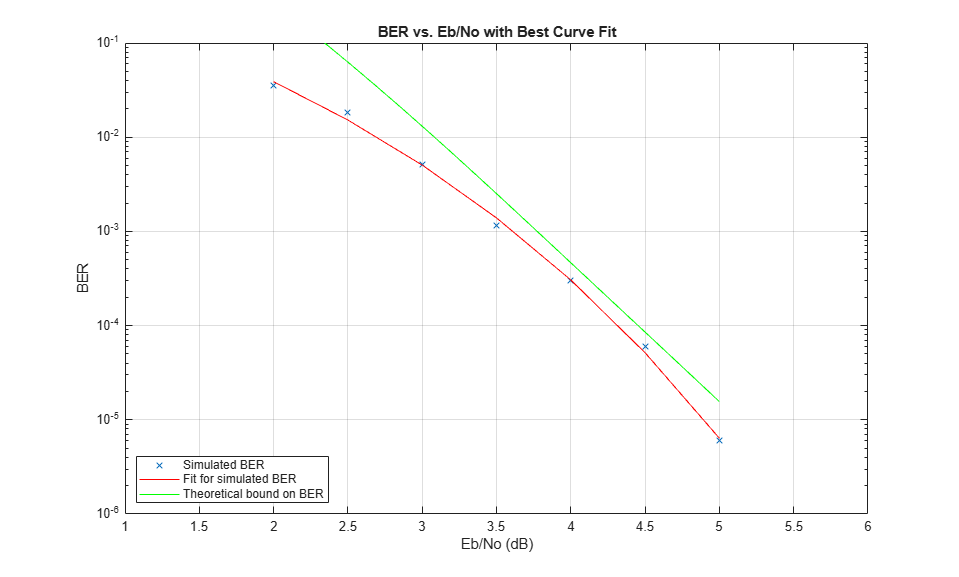In some cases, at the lower bit error rates, you might notice simulation results that appear to indicate error rates slightly above the bound. This result can come from the finite traceback depth in the decoder or, if you observe fewer than 500 bit errors, from simulation variance.

For an example that shows convolutional coding without puncturing, see the Soft-Decision Decoding section in Error Detection and Correction.

References

1. Yasuda, Y., K. Kashiki, and Y. Hirata, "High Rate Punctured Convolutional Codes for Soft Decision Viterbi Decoding," IEEE Transactions on Communications, Vol. COM-32, March, 1984, pp. 315–319.

2. Begin, G., Haccoun, D., and Paquin, C., "Further results on High-Rate Punctured Convolutional Codes for Viterbi and Sequential Decoding," IEEE Transactions on Communications, Vol. 38, No. 11, November, 1990, p. 1923.

Use the Viterbi Decoder block for fixed-point hard- and soft-decision convolutional decoding. Compare results to theoretical upper bounds as computed with the Bit Error Rate Analysis app.

Simulation Configuration

The cm_viterbi_harddec_fixpt and cm_viterbi_softdec_fixpt models highlight the fixed-point modeling attributes of the Viterbi decoder, using similiar layouts. The default configuration of the models use the PreLoadFcn Callback to specify ansetting of 4 dB for the AWGN Channel block. The convolutional encoder is configured as a rate 1/2 encoder. Specifically, for every 2 bits the encoder adds another 2 redundant bits. To accommodate the code rate, the Eb/No (dB) parameter of the AWGN block is halved by subtracting 10*log10(2) from the assignedsetting. To limit the run duration to 100 errors or 1e6 bits for the Error Rate Calculation block.

Fixed-Point Modeling

Fixed-point modeling enables bit-true simulations which take into account hardware implementation considerations and the dynamic range of the data and parameters. For example, if the target hardware is a DSP microprocessor, some of the possible word lengths are 8, 16, or 32 bits, whereas if the target hardware is an ASIC or FPGA, there may be more flexibility in the word length selection.

To enable fixed-point Viterbi decoding,

• For hard decisions, the block input must be of type ufix1 (unsigned integer of word length 1). Based on this input (either a 0 or a 1), the internal branch metrics are calculated using an unsigned integer of word length = (number of output bits), as specified by the trellis structure (which equals 2 for the hard-decision example).

• For soft decisions, the block input must be of type ufixN (unsigned integer of word length N), where N is the number of soft-decision bits, to enable fixed-point decoding. The block inputs must be integers in the range 0 to. The internal branch metrics are calculated using an unsigned integer of word length = (N + number of output bits - 1), as specified by the trellis structure (which equals 4 for the soft-decision example).

The State metric word length is specified by the user and usually must be greater than the branch metric word length already calculated. You can tune this to be the most suitable value (based on hardware and data considerations) by reviewing the logged data for the system.

Enable the logging by selecting Apps > Fixed-Point Tool. In the Fixed-Point Setting menu, set the Fixed-point instruments mode to Minimums, maximums and overflows, and rerun the simulation. If you see overflows, it implies the data did not fit in the selected container. You could either try scaling the data prior to processing it or, if your hardware allows, increase the size of the word length. Based on the minimum and maximum values of the data, you are also able to determine whether the selected container is of the appropriate size.

Try running simulations with different values of State metric word length to get an idea of its effect on the algorithm. You should be able to narrow down the parameter to a suitable value that has no adverse effect on the BER results.

The hard decision configuration for the cm_viterbi_harddec_fixpt model:• The BPSK Demodulator Baseband produces hard decisions, which are passed onto the decoder.

• The Data Type Conversion (Simulink) block sets the Mode parameter to Fixed point and casts the output data type to fixdt(0,1,0). The signal input to the Viterbi Decoder block is ufix1.

• The Viterbi Decoder block has the Decision type parameter set to Hard decision, and on the Data Types tab, the State metric word length is set to 4 and the Output data type is set to boolean. The bit error rate is displayed and captured to the BER workspace variable.

The soft decision configuration for the cm_viterbi_softdec_fixpt model:• The Viterbi Decoder block has the Decision type parameter set to Soft decision and Number of soft decision bits set to 3, and on the Data Types tab, the State metric word length is set to 6 and the Output data type is set to boolean. The bit error rate is displayed and captured to the BER workspace variable.

Comparisons Between Hard and Soft-Decision Decoding

The two models are configured to run from within the Bit Error Rate Analysis app to generate simulation curves to compare the BER performance for hard-decision versus soft-decision decoding.

You can produce the results in this plot by following the steps outlined below.These steps generate simulation results for theoretical and fixed-point hard and soft decision Viterbi decoding:

1. Open the Bit Error Rate Analysis app by selecting it in the Apps tab or by entering bertool at the MATLAB command prompt.

2. On the Theoretical pane, with Eb/N0 range set to 2:5, Channel type set to AWGN, Modulation type set to PSK, Channel coding set to Convolutional, run with Decision method set to Hard and then Soft. Rename the BER Data Set to identify the hard and soft data sets of theoretical results.

3. On the Monte Carlo pane, set Eb/N0 range to 2:1:5, for Simulation environment select Simulink, BER variable name set to BER, for Simulation limits set Number of errors to 100 and Number of bits to 1e6. Run with Model name set to cm_viterbi_harddec_fixpt and then cm_viterbi_softdec_fixpt. Rename the BER Data Set to identify the hard and soft data sets of Simulink results.

After the four runs the app will resemble this image.Comparisons with Double-Precision Data

For further explorations, you can run the same model with double precision data by selecting Apps > Fixed-Point Tool. In the Fixed-Point Tool app, select the Data type override to be Double. This selection overrides all data type settings in all the blocks to use double precision. For the Viterbi Decoder block, as Output type was set to boolean, this parameter should also be set to double.

Upon simulating the model, note that the double-precision and fixed-point BER results are the same. They are the same because the fixed-point parameters for the model have been selected to avoid any loss of precision and optimize memory efficiency.

## Ports

### Input

expand all

Convolutionally encoded codeword, specified as a column vector. If the decoder takes N input bit streams (that is, it can receive 2N possible input symbols), the block input vector length is L×N for some positive integer L. For more information, see Input and Output Sizes, Input Values and Decision Types, and the Operation mode parameter.

This port is unnamed until a second input port is enabled.

Data Types: double | single | Boolean | int8 | int16 | int32 | uint8 | uint16 | uint32 | ufixn

Erasure bits in the codeword, specified as a binary-valued vector. Values of 1 in the vector correspond to erased bits, and values of 0 correspond to nonerased bits.

For these erasures in the incoming data stream, the decoder does not update the branch metric. The widths and the sample times of the erasure and the input data ports must be the same.

#### Dependencies

To enable this port, select Enable erasures input port.

Data Types: double | Boolean

Option to reset the state of decoder registers, specified as scalar value. When this port receives a nonzero input value, the block sets its internal memory to the initial state before processing the input data. Resetting the decoder registers to the initial state sets:

• The all-zeros state metric to zero

• All other state metrics to the maximum value

• The traceback memory to zero

Using the reset port on this block is analogous to setting Operation mode for the Convolutional Encoder block to Reset on nonzero input via port.

#### Dependencies

To enable this port, set the Operation mode parameter to Continuous and select Enable reset input port.

Data Types: double | Boolean

### Output

expand all

Output message, returned as a binary column vector. If the decoder produces K output bit streams (that is, it can produce 2K possible output symbols), the block output vector length is L×K for some positive integer L. For more information, see Input and Output Sizes.

This port is unnamed on the block icon.

Data Types: double | single | Boolean | int8 | int16 | int32 | uint8 | uint16 | uint32 | ufix1

## Parameters

expand all

To edit block parameters interactively, use the Property Inspector. From the Simulink® Toolstrip, on the Simulation tab, in the Prepare gallery, select .

Main

Trellis description of the convolutional code, specified as a structure that contains the trellis description for a rate KN code. K is the number of input bit streams, and N is the number of output bit streams.

You can either use the poly2trellis function to create the trellis structure or create it manually. For more about this structure, see Trellis Description of a Convolutional Code and the istrellis function.

The trellis structure contains these fields.

Number of symbols input to the encoder, specified as an integer equal to 2K, where K is the number of input bit streams.

Number of symbols output from the encoder, specified as an integer equal to 2N, where N is the number of output bit streams.

Number of states in the encoder, specified as a power of 2.

Next states for all combinations of current states and current inputs, specified as a matrix of integers. The matrix size must be numStates by 2K.

Outputs for all combinations of current states and current inputs, specified as a matrix of octal numbers. The matrix size must be numStates by 2K.

Select this parameter to view and enable the Puncture vector parameter.

Puncture pattern vector to puncture the decoded data, specified as a column vector. The vector must contain 1s and 0s, where 0 indicates the position of the punctured bits. This puncture pattern must match the puncture pattern used by the convolutional encoder.

For some commonly used puncture patterns for specific rates and polynomials, see the Yasuda , Haccoun , and Begin  references.

#### Dependencies

This parameter appears only when you select the Punctured code parameter.

Select this parameter to add the Era input port to the block.

Decoder decision type, specified as Unquantized, Hard decision, or Soft Decision.

• Unquantized — The decoder uses the Euclidean distance to calculate the branch metrics. The input data must be a real-valued vector of double- or single-precision soft values that are unquantized. The object maps positive values to logical 1s and negative values to logical 0s

• Hard decision — The decoder uses Hamming distance to calculate the branch metrics. The input must be a vector of hard decision values, which are 0s or 1s. The data type of the inputs must be double precision, single precision, logical, or numeric.

• Soft Decision — The decoder uses Hamming distance to calculate the branch metrics. The input requires a vector of quantized soft values that are represented as integers between 0 and 2SoftInputWordLength – 1. The data type of the inputs must be double precision, single precision, logical, or numeric. Alternatively, you can specify the data type as an unsigned and unscaled fixed-point object using the fi (Fixed-Point Designer) object with a word length (SoftInputWordLength) equal to the word length that you specify for the Number of soft decision bits parameter. 0 is considered as most confident 0 and 2SoftInputWordLength – 1 as the most confident 1.

Soft input word length that represents the number of bits for each quantized soft input value, specified as an integer.

#### Dependencies

This parameter appears only when you set the Decision type parameter to Soft decision.

Select this parameter to error if the quantized input values are out of range. If you do not select this parameter, out of range input values are ignored.

#### Dependencies

This parameter appears only when you set the Decision type parameter to Hard decision or Soft decision.

Traceback depth, specified as an integer that indicates the number of trellis branches used to construct each traceback path.

The traceback depth influences the decoding delay. The decoding delay is the number of zero symbols that precede the first decoded symbol in the output.

• For the continuous operating mode, the decoding delay is equal to the number of traceback depth symbols.

• For the truncated or terminated operating mode, the decoding delay is zero. In this case, the traceback depth must be less than or equal to the number of symbols in each input.

As a general estimate, a typical traceback depth value is approximately two to three times (ConstraintLength – 1) / (1 – coderate). The constraint length of the code, ConstraintLength, is equal to (log2(trellis.numStates) + 1). The coderate is equal to (K / N) × (length(PuncturePattern) / sum(PuncturePattern).

K is the number of input symbols, N is the number of output symbols, and PuncturePattern is the puncture pattern vector.

For example, applying this general estimate, results in these approximate traceback depths.

• A rate 1/2 code has a traceback depth of 5(ConstraintLength – 1).

• A rate 2/3 code has a traceback depth of 7.5(ConstraintLength – 1).

• A rate 3/4 code has a traceback depth of 10(ConstraintLength – 1).

• A rate 5/6 code has a traceback depth of 15(ConstraintLength – 1).

Method for transitioning between successive input frames, specified as one of these mode values.

• Continuous — The block saves its internal state metric at the end of each input, for use with the next frame. Each traceback path is treated independently. This mode results in a decoding delay of Traceback depth×K zero bits for a rate K/N convolutional code. K is the number of message symbols, and N is the number of coded symbols. If the Enable reset input port is selected, the decoder states are reset if the Rst port receives a nonzero value.

• Truncated — The block treats each input independently. The traceback path starts at the state with the best metric and always ends in all-zeros state. This mode is appropriate when the corresponding Convolutional Encoder block has its Operation mode set to Truncated (reset every frame). There is no output delay for this mode.

• Terminated — The block treats each input independently, and the traceback path always starts and ends in all-zeros state. This mode is appropriate when the uncoded message signal (that is, the input to the corresponding Convolutional Encoder block) has enough zeros at the end of each input to fill all memory registers of the feed-forward encoder. Specifically, there are at least k*max(constr-1) zeros at the end of the input, for an encoder that has k input streams and constraint length vector constr (using the polynomial description). For feedback encoders, this mode is appropriate if the corresponding Convolutional Encoder block has Operation mode set to Terminate trellis by appending bits.

Note

The decoder states reset at every input time step when the block outputs sequences that vary in length during simulation and you set the Operation mode to Truncated or Terminated.

When the input signal contains only one symbol, use the Continuous operation mode.

Select this parameter to add the Rst input port.

#### Dependencies

This parameter appears only when you set the Operation mode parameter to Continuous.

Select this parameter to delay reset of the decoder until after computing the encoded data received in the current time step. You must enable this option for HDL support. Generating HDL code requires HDL Coder™ software.

#### Dependencies

This parameter appears only when you set the Operation mode parameter to Continuous and select Enable reset input port.

Data Types

State metric word length, specified as a positive integer.

Output data type, specified as double, single, boolean, int8, uint8, int16, uint16, int32, or uint32, or set to 'Inherit via internal rule' or 'Smallest unsigned integer'.

• When set to 'Smallest unsigned integer', the output data type is selected based on the settings used in the Hardware Implementation pane of the Configuration Parameters dialog box of the model. If ASIC/FPGA is selected in the Hardware Implementation pane, the output data type is ufix(1). For all other selections, it is an unsigned integer with the smallest specified word length corresponding to the char value (for example, uint8).

• When set to 'Inherit via internal rule', the block:

• Outputs data type double for double inputs

• Outputs data type single for single inputs

• Behaves similar to the 'Smallest unsigned integer' option for all other data type inputs

## Block Characteristics

 Data Types Boolean | double | fixed pointa | integer | single Multidimensional Signals no Variable-Size Signals yes a Input can be ufix(1) for hard decision, and ufix(N) for soft decision; output can be ufix(1) only.

expand all

 Clark, George C., and J. Bibb Cain. Error-Correction Coding for Digital Communications. Applications of Communications Theory. New York: Plenum Press, 1981.

 Gitlin, Richard D., Jeremiah F. Hayes, and Stephen B. Weinstein. Data Communications Principles. Applications of Communications Theory. New York: Plenum Press, 1992.

 Heller, J., and I. Jacobs. “Viterbi Decoding for Satellite and Space Communication.” IEEE Transactions on Communication Technology 19, no. 5 (October 1971): 835–48. https://doi.org/10.1109/TCOM.1971.1090711.

 Yasuda, Y., K. Kashiki, and Y. Hirata. “High-Rate Punctured Convolutional Codes for Soft Decision Viterbi Decoding.” IEEE Transactions on Communications 32, no. 3 (March 1984): 315–19. https://doi.org/10.1109/TCOM.1984.1096047.

 Haccoun, D., and G. Begin. “High-Rate Punctured Convolutional Codes for Viterbi and Sequential Decoding.” IEEE Transactions on Communications 37, no. 11 (November 1989): 1113–25. https://doi.org/10.1109/26.46505.

 Begin, G., D. Haccoun, and C. Paquin. “Further Results on High-Rate Punctured Convolutional Codes for Viterbi and Sequential Decoding.” IEEE Transactions on Communications 38, no. 11 (November 1990): 1922–28. https://doi.org/10.1109/26.61470.

 Moision, B. "A Truncation Depth Rule of Thumb for Convolutional Codes." In Information Theory and Applications Workshop (January 27 2008-February 1 2008, San Diego, California), 555-557. New York: IEEE, 2008.